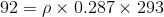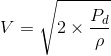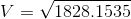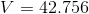# The air velocity in a duct is measured by a Pitot-static probe connected to a differential pressure gage. If the air is at 92 kPa absolute and 20°C and the reading of the differential pressure gage is 1.0 kPa, determine the air velocity.

Question-AnswerCategory: Fluid MechanicsThe air velocity in a duct is measured by a Pitot-static probe connected to a differential pressure gage. If the air is at 92 kPa absolute and 20°C and the reading of the differential pressure gage is 1.0 kPa, determine the air velocity.

The air velocity in a duct is measured by a Pitot-static probe connected to a differential pressure gage. If the air is at 92 kPa absolute and 20°C and the reading of the differential pressure gage is 1.0 kPa, determine the air velocity.

Given,
Absolute pressure of Air (P) = 92 kPa
Absolute Temperature (T) = 20o C = 20+273 = 293 K
Differential Pressure gage (Pd) = 1.0 kPa

We can use ideal gas equation to find density of Air in duct
PV = mRT
P = ρRT ………(1)                 [  ρ = (m/V) ]
P = Absolute Pressure
ρ = density
R = Characteristic Gas Constant
= 0.287 kJ/kg-K  (for AIR)
T = Temperature in Kelvin
Putting the values in equation (1)For Pitot Static we know the Formula for velocity is……..(2)
V = Velocity of air in duct
ρ = density of air
Pd = Differential pressure gage = 1.0 kPa = 1000 Pa
putting values in equation (2)